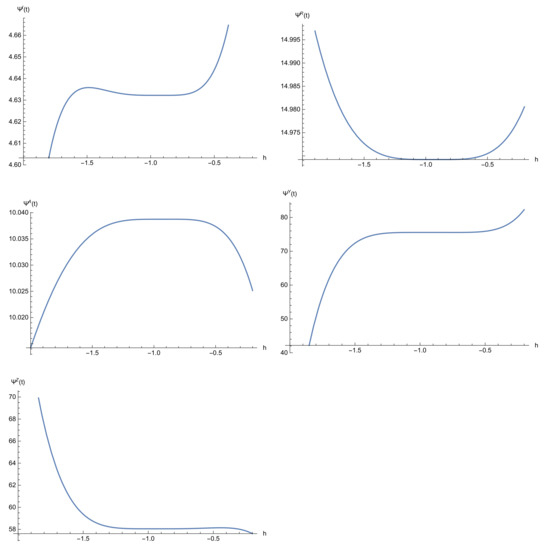Next Article in Journal
Multi-Output Learning Based on Multimodal GCN and Co-Attention for Image Aesthetics and Emotion Analysis
Next Article in Special Issue
Expert System to Model and Forecast Time Series of Epidemiological Counts with Applications to COVID-19
Previous Article in Journal
Quasistatic Porous-Thermoelastic Problems: An a Priori Error Analysis
Previous Article in Special Issue
Using a Modified VIKOR Technique for Evaluating and Improving the National Healthcare System Quality

Article

# A Comparative Study between Discrete Stochastic Arithmetic and Floating-Point Arithmetic to Validate the Results of Fractional Order Model of Malaria Infection

1
Department of Applied Mathematics and Programming, South Ural State University, Lenin Prospect 76, 454080 Chelyabinsk, Russia
2
Industrial Mathematics Laboratory, Baikal School of BRICS, Irkutsk National Research Technical University, 664074 Irkutsk, Russia
3
Department of Engineering Science, Bandırma Onyedi Eylül University, Bandırma 10200, Balıkesir, Turkey
4
*
Author to whom correspondence should be addressed.
Academic Editors: Victoria Lópezs and Laureano González Vega
Mathematics 2021, 9(12), 1435; https://doi.org/10.3390/math9121435
Received: 8 May 2021 / Revised: 15 June 2021 / Accepted: 17 June 2021 / Published: 20 June 2021
(This article belongs to the Special Issue Mathematical Modeling and Its Application in Medicine)
The researchers aimed to study the nonlinear fractional order model of malaria infection based on the Caputo-Fabrizio fractional derivative. The homotopy analysis transform method (HATM) is applied based on the floating-point arithmetic (FPA) and the discrete stochastic arithmetic (DSA). In the FPA, to show the accuracy of the method we use the absolute error which depends on the exact solution and a positive value $\epsilon$. Because in real life problems we do not have the exact solution and the optimal value of $\epsilon$, we need to introduce a new condition and arithmetic to show the efficiency of the method. Thus the CESTAC (Controle et Estimation Stochastique des Arrondis de Calculs) method and the CADNA (Control of Accuracy and Debugging for Numerical Applications) library are applied. The CESTAC method is based on the DSA. Also, a new termination criterion is used which is based on two successive approximations. Using the CESTAC method we can find the optimal approximation, the optimal error and the optimal iteration of the method. The main theorem of the CESTAC method is proved to show that the number of common significant digits (NCSDs) between two successive approximations are almost equal to the NCSDs of the exact and approximate solutions. Plotting several graphs, the regions of convergence are demonstrated for different number of iterations k = 5, 10. The numerical results based on the simulated data show the advantages of the DSA in comparison with the FPA. View Full-Text
Show FiguresFigure 1

MDPI and ACS Style

Noeiaghdam, S.; Dreglea, A.; Işık, H.; Suleman, M. A Comparative Study between Discrete Stochastic Arithmetic and Floating-Point Arithmetic to Validate the Results of Fractional Order Model of Malaria Infection. Mathematics 2021, 9, 1435. https://doi.org/10.3390/math9121435

AMA Style

Noeiaghdam S, Dreglea A, Işık H, Suleman M. A Comparative Study between Discrete Stochastic Arithmetic and Floating-Point Arithmetic to Validate the Results of Fractional Order Model of Malaria Infection. Mathematics. 2021; 9(12):1435. https://doi.org/10.3390/math9121435

Chicago/Turabian Style

Noeiaghdam, Samad, Aliona Dreglea, Hüseyin Işık, and Muhammad Suleman. 2021. "A Comparative Study between Discrete Stochastic Arithmetic and Floating-Point Arithmetic to Validate the Results of Fractional Order Model of Malaria Infection" Mathematics 9, no. 12: 1435. https://doi.org/10.3390/math9121435

Find Other Styles
Note that from the first issue of 2016, MDPI journals use article numbers instead of page numbers. See further details here.

1